#Quality Digest

Featured Product
This Week in Quality Digest Live
Lean Features
Del Williams
8-in. cable and disc systems are comparable to belt or bucket systems
Jeremy L. Boerger
Kevin Ketels
The baby formula industry was primed for disaster long before a key factory closed down
Joe Vernon
The greatest advantage of CV is its ability to count and categorize inventory
James J. Kline
Quality professional organizations need to adjust their body of knowledge to include an understanding of big data
Lean News
Sept. 28–29, 2022, at the MassMutual Center in Springfield, MA
Enables system-level modeling with 2D and 3D visualization, reducing engineering effort, risk, and cost
It is a smart way to eliminate waste and maximize value
Simplified process focuses on the fundamentals every new ERP user needs
DigiLEAN software helps companies digitize their lean journey
Partnership embeds quality assurance at every stage of the product life cycle, enables agile product introduction
First trial module of learning tool focuses on ISO 9001 and is available now
Offset-aware programming of spindle transfers and bar pulls helps manufacturers drive multichannel CNC machinery
Freedom platform connects to any industrial asset to provide automated intelligence related to asset availability, utilization, and continuous improvementBio

Lean

## Short Run SPC, Part 4

### Standardized charts for subgrouped data

Published: Monday, March 2, 2020 - 13:03

All articles in this series:

Lean production is built on the explicit assumption that each step is operated predictably and capably. Predictable operation can only be achieved and maintained by using process behavior charts. But short production runs make it hard to see how to use process behavior charts. Parts one and two illustrated how to chart individual values. This paper will illustrate the Zed-Bar chart for use with subgrouped data.

### Subgrouped data

When we subgroup values together we are making an explicit assumption that the values within each subgroup were collected under essentially the same conditions. This immediately implies two things: first, that no useful information is lost by averaging the values together, and second, that the differences within the subgroups represent the inherent routine process variation. Hence, subgrouping always requires a judgment call based on the context. It is the internal homogeneity of the subgroups that makes ordinary average and range charts work, and this also holds true for Zed-Bar charts.

When several consecutive parts, or nearly consecutive parts, are collected at specified intervals of time, then subgrouping makes sense. However, when the data consist of single values spaced out over time, subgrouping may, or may not, make sense depending on the context.

With short production runs we are likely to have limited amounts of data for each product. If a short production run gives us four values for Product A, we can either plot these four points on a zed chart, or we can combine them and plot one average on a Zed-Bar chart. With a zed chart each of the four points can be used for feedback by the operator. With a Zed-Bar chart the operator ends up with a report-card value for Product A after the short run is over.

So, unless the context provides a good reason for subgrouping, I recommend plotting individual values with short-run data. However, when you have data which logically belongs in subgroups, and when those subgroups are all the same size, the Zed-Bar chart will let you combine subgroups for different products on the same chart.

### Unit 15

Three products are produced in short runs on Unit 15. Samples of five pieces are collected at the start of each run and periodically thereafter throughout the run. Since the runs are of different lengths they usually end up with two, three, or four subgroups for each product run.

Product A was produced in Runs 1, 3, 4, and 6. Product B was produced in Runs 2 and 7. And Product C was produced in Runs 5 and 8. As always we begin with the product specific charts. In this case they are average and range charts with subgroup size 5. The data are included with each chart.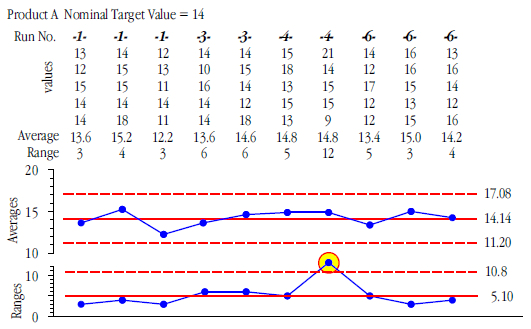Figure 1: Average and range chart for Product A

Product A has a target value of 14, a grand average of 14.14, and an average range of 5.10. Except for the second subgroup of Run 4, they seem to be doing a fairly good job of operating on target.Figure 2: Average and range chart for Product B

Product B has a target value of 40, a grand average of 40.60, and an average range of 9.167. While we can see a difference between the Run 2 and Run 7, we do not have quite enough data to say that this is a detectable difference.Figure 3: Average and range chart for Product C

Product C has a target value of 28, a grand average of 26.65, and an average range of 6.00. Except for the first subgroup of Run 5 they seem to have been operating in the neighborhood of 28.

These product-specific average and range charts set the stage for creating a standardized average and range chart that will allow real-time tracking of the production process following the baseline period.

(Those who may have access to my book Short Run SPC (SPC Press) should note that the notation used below is different from the notation in chapter six of that book.)

### Zed-Bar charts

There are several different, but fully equivalent, versions of the standardized average and range chart. We begin with the simplest version, the Zed-Bar and W chart. This version of the standardized average and range chart transforms the subgroup averages and subgroup ranges using:Where both the nominal value and the average range are specific to the product being measured in that subgroup. As before, the nominal value for a product may be either a target value or the baseline grand average value for that product.

The limits for subgroup averages and subgroup ranges are commonly expressed as:(The scaling factors above may be found in figure 11.) When we subtract off the grand average (or a target value) we are effectively centering the limits for the subgroup averages on zero. When we divide by the average range we are changing the scale of the limits. Thus, the limits for the Zed-Bar and W chart become:When the subgroup size is smaller than seven the value for D3 is negative and there will be no lower limit for the W values.

We will use the data from figures 1, 2, and 3 to illustrate this standardized average and range chart. In this example we will use the target values as the nominals.

### Zed-Bar chart for Unit 15

For the Product A subgroups, Nominal(A) = 14 and the average range is R-bar(A) = 5.10, hence the transforms used for Product A averages and ranges are:For the Product B subgroups, Nominal(B) = 40 and R-bar(B) = 9.167, hence the transforms used for Product B averages and ranges are:And for Product C subgroups, Nominal(C) = 28 and R-bar(C) = 6.00, hence the transforms used for Product C averages and ranges are:When the subgroup averages and ranges from figures 1, 2, and 3 are placed in production run order and transformed as above, we get our Zed-Bar and W chart in figure 4. With subgroups of size n = 5 the Zed-Bar limits are  ± A2 = ± 0.577. The W chart has a central line of 1.00, an upper limit of D4 = 2.114, and no lower limit.Figure 4: Zed-Bar and W chart for Unit 15

The two points outside the limits in figure 4 may also be seen in figures 1 and 3. Here we see that they occurred on successive runs which raises the question of whether these two signals might be related in some way.  Except for these two points, the rest of the subgroups Unit 15 seem to have been reasonably close to their target values.

As future production runs of these three products occur the transformed subgroup averages and ranges may be placed on the extension of figure 4. In this way the process may be visualized in real time while it is used to make different products.

In this version of the standardized average and range chart we used the average range to “standardize” the values plotted, and the limits are given by the standard scaling factors for average and range charts. Thus, a simple division transforms the original averages and ranges into values that allow different products to be placed on the same chart.

### Version two: Zed-Bar*charts

In the second version of the standardized average and range chart we use a different denominator in our transformations. Instead of the average range we use Sigma(X)

Sigma(X) is a generic notation for any within-subgroup estimator of the standard deviation for the original values. In the context of an average and range chart Sigma(X) will generally refer to:Where d2 is the bias correction factor for subgroups of size n (see figure 11). Thus:Since we can find these standardized values by multiplying the earlier Zed-Bar and W values by the bias correction factor d2, we denote these version two standardized values by Zed-Bar* and W*.

This also shows us how to find the limits for Zed-Bar* and W*. We multiply the limits for version one by d2. Hence:Where d2 and d3 are the standard bias correction factors for ranges from subgroups of size n

For Unit 15 the subgroup size was n = 5, so d2 = 2.326, and d3 = 0.8641. Thus, the limits for the Zed-Bar* chart become 2.326 (±0.577)  = ±1.34, and the W* chart has a central line of 2.326 and an upper limit of 4.918. When the original subgroup averages and ranges for each of the products are transformed with the Zed-Bar* and W* formulas we get figure 5.Figure 5: Zed-Bar* and W* chart for Unit 15

The only difference between figure 4 and figure 5 is that they have different vertical scales. While the values plotted are numerically different, the limits change by the same amount, and point by point the story remains the same.

### Version three: Zed-Bar** charts

A third version of the standardized average chart uses yet another denominator for standardizing the subgroup averages. Here we use a within-subgroup estimate of the standard deviation for the subgroup averages, which we shall denote as Sigma(X-bar). When working with average and range charts this estimate is generally taken to be: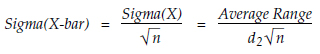When we standardize the subgroup averages using Sigma(X-bar) we end up with the Zed-Bar** values.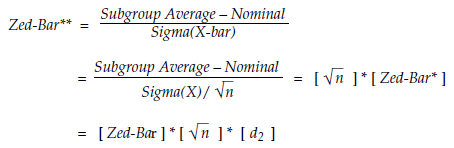Thus, Zed-Bar** is simply the original Zed-Bar value multiplied by both a bias correction factor and the square root of the subgroup size. Hence we label it as Zed-Bar**.

The limits for Zed-Bar** become: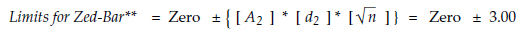These limits are completely independent of the subgroup size since the subgroup size is taken into account in the transformation of the subgroup averages.

There is no point in dividing the subgroup ranges by Sigma(X-bar) because Sigma(X) fully standardizes the subgroup ranges. Instead, we commonly use W* (the standardized ranges from Version Two) along with Zed-Bar**.

For Unit 15 the limits for the Zed-Bar** chart become ±3.00, and the W* chart remains the same as in figure 5, with a central line of 2.326 and an upper limit of 4.918. When the original subgroup averages and ranges are transformed with the Zed-Bar** and W* formulas we get figure 6.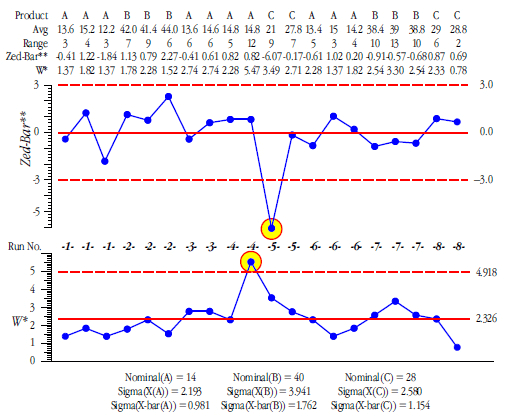Figure 6: Zed-Bar** and W* chart for Unit 15

While the numerical values that are plotted in figures 4, 5, and 6 change, the limits change with them so that the picture remains the same. The three versions simply use different scales. Given this equivalence, the simplicity of the first version is hard to resist (where we divided everything by the appropriate average range, and used the usual scaling factors as limits). Figures 5 and 6 merely add levels of complexity that serve no real purpose.

### When the nominal is the grand average

When creating a standardized chart the user gets to pick the nominal value. When the nominal values are the target values the standardized chart tracks how closely the process is operated on-target. When the nominal values are the baseline grand average for each product, the standardized chart will track how well the future production runs match up with the baseline runs.

For Unit 15, Product A has a grand average of 14.14 and a target value of 14. Regardless of which value we use as the nominal, we will get pretty much the same standardized values for Product A.

Product B has a grand average of 40.60 and a target value of 40. Subtracting off a larger value will shift the standardized average values for Product B down slightly compared with figure 4.

Product C has a grand average of 26.65 and a target value of 28. Here when we use the grand average as the nominal we will get standardized average values that are shifted up relative to figure 4.

The Zed-Bar and W chart based on the grand averages as nominals is shown in figure 7. The white dots show the Zed-Bar values from figure 4 for comparison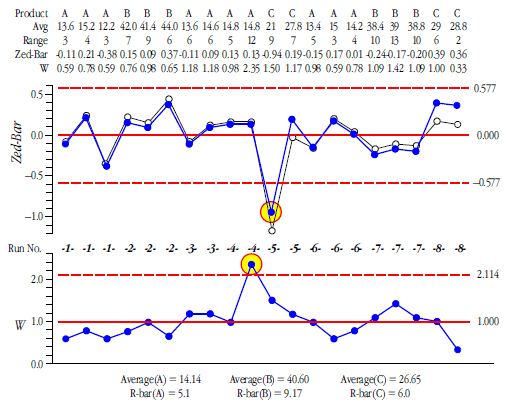Figure 7: Zed-Bar and W chart for Unit 15 with nominals = grand averages

The choice between a target value and a grand average for the nominal value should be based upon the context for the process being tracked and how you want to use the chart. Here both charts tell the same story because they were (mostly) doing a reasonable job of operating near the target values.

These three versions of a standardized average and range chart are summarized in figure 8.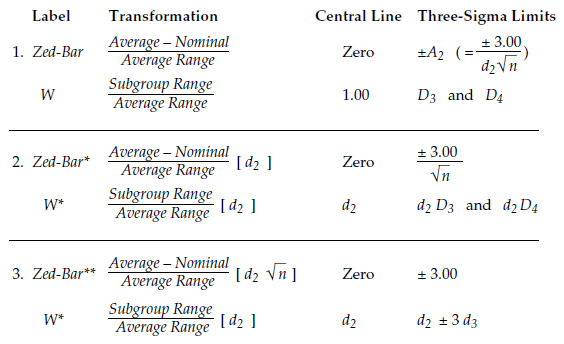Figure 8: Three versions of the standardized average and range chart

### Version four: Standardized average and standard deviation charts

In the same way that we standardized the average and range chart we can define Zed-Bar and S charts based on average and standard deviation charts. The Zed-Bar and S chart transforms the subgroup averages and subgroup standard deviation statistics using:The limits for the Zed-Bar and S chart are:Where c4 is the bias correction factor for subgroup standard deviation statistics from subgroups of size n.

For Product A subgroups, Nominal(A) = 14 and s-bar(A) = 2.00, hence the transforms used for Product A averages and standard deviations would be: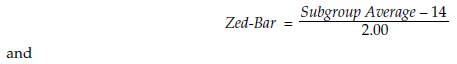For Product B subgroups, Nominal(B) = 40 and s-bar(B) = 3.56, hence the transforms used for Product B averages and standard deviations would be: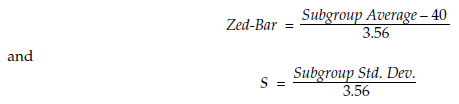And for Product C subgroups, Nominal(C) = 28 and s-bar(C) = 2.67, hence the transforms used for Product C averages and standard deviations would be: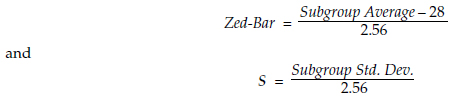When the subgroups are placed in production order and transformed as above, the Zed-Bar chart will have a central line of zero and limits of ±A3 where A3 is the usual scaling factor for an average chart for subgroups of size n. With subgroups of size n = 5 the value for A3 is 1.427.

The S chart will have a central line of 1.00 and limits of B3 and B4. For subgroups of size n = 5, B4 = 2.089 and there is no lower limit. Figure 8 shows the Zed-Bar and S chart for Unit 15.Figure 9: Zed-Bar and S chart for Unit 15

The white dots show the equivalent running record from figure 4 for the sake of comparison. Figure 8 tells the same story as figures 4, 5, 6, and 7. This happens because the subgroup ranges and subgroup standard deviations are always highly correlated for subgroup sizes of 15 or less. With subgroups of size five the ranges and standard deviation statistics always have a correlation coefficient of 97.5 percent; hence it makes virtually no difference whether we use an average and range chart or an average and standard deviation chart.

Of course, we can also define additional versions of the standardized average and standard deviation chart in the manner done before. When we do this we end up with the limits shown in figure 10 for these various versions.Figure 10: Three versions of standardized average and standard deviation charts

### Working with software

When working with software that attempts to present a standardized average and range chart you might find any one of the six versions above. In practice you can usually determine which transformation has been used by looking at the values for the limits on the chart.

If you are unable to puzzle it out directly, you may evaluate your software by using the data given here. If you do this and do not find the equivalent of figure 4, 5, 6, 7, or 8, your software may be standardizing the data in some incorrect or inappropriate manner (e.g., as covered in Short Run SPC, Part 3).

### How many data

The quality of a Zed-Bar chart will depend upon how many data are used for each product. When using fewer than 12 observations the statistics used will be soft and uncertain. With 12 to 33 observations the statistics will gel and solidify. Once we have more than 33 values in the baseline for a product our limits will be solid enough that additional data will make little difference in the resulting charts.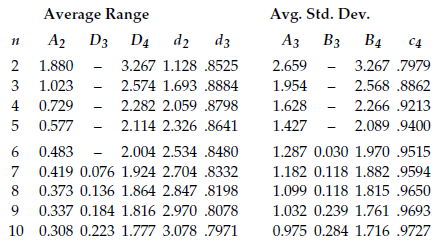Figure 11: Scaling factors and bias correction factors for Zed-Bar charts

### Recommendations

Whenever the data are limited or when the data are collected periodically you should always begin with charts for individual values. If the process tends to change slowly relative to the sample frequency, then such data may be subgrouped in appropriate ways with little loss of information.

When the data are obtained in clusters, the subgrouping of these data and the use of Zed-Bar charts may be appropriate. If the chart is to be used by those running the process one should be careful to avoid subgrouping too much data together. With large subgroup sizes, or a low subgroup frequency, short production runs can result in charts that are nothing but ancient history. With short production runs it is often better to measure fewer parts more frequently and to use charts for individual values, than it is to subgroup larger amounts of data that are obtained less often.

### Discuss